# C++ program to find even and odd elements in array

In this tutorial you will learn about the C++ program to find even and odd elements in array and its application with practical example.

## C++ program to find even and odd elements in the array

In this tutorial, we will learn to create a C++ program that will find even and odd elements in the array using C++ programming.

## Prerequisites

Before starting with this tutorial we assume that you are best aware of the following C++ programming topics:

• Operators in C++ Programming.
• Basic Input and Output function in C++ Programming.
• Basic C++ programming.
• For loop in C++ programming.
• Conditional Statements in C++ programming.
• Arithmetic operations in C++ Programming.

## Even and Odd Numbers:-

The Even numbers are those numbers that are completely divisible by 2. which means the reminder should be zero. And the Odd numbers are those numbers that are not completely divisible by 2.

## Program to find even and odd elements in the array

In C++ programming, it is possible to take integer input from the user and find even and odd elements in the array with the help of a very small amount of code. We will pass that array to a condition to find even and odd numbers from the array.

With the help of the below program, we can Find Even & Odd elements in the array.

## Output:-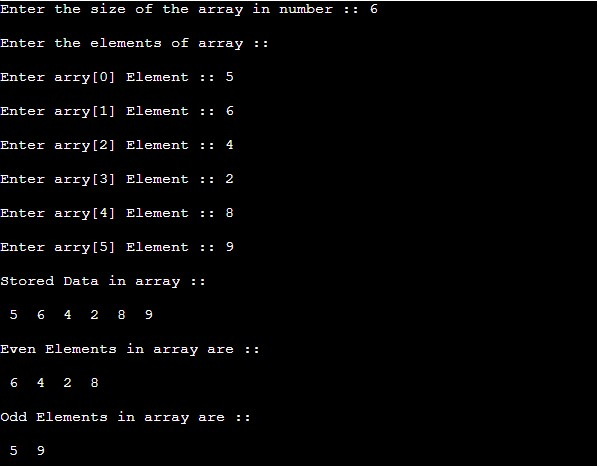In the above program, we have first initialized the required variable.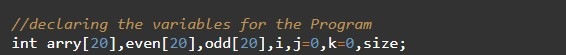• arry = it will hold the integer value.
• even = it will hold the integer value.
• odd = it will hold the integer value.
• i = it will hold the integer value.
• j = it will hold the integer value.
• k = it will hold the integer value.
• size = it will hold the integer value.
Taking input from the user the size and the elements of the array.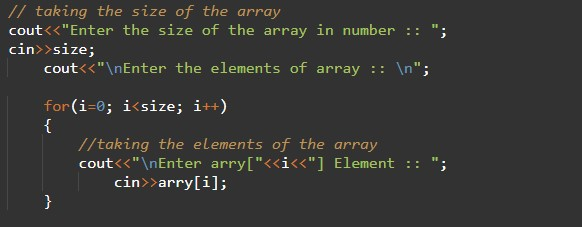Stored Data in the array.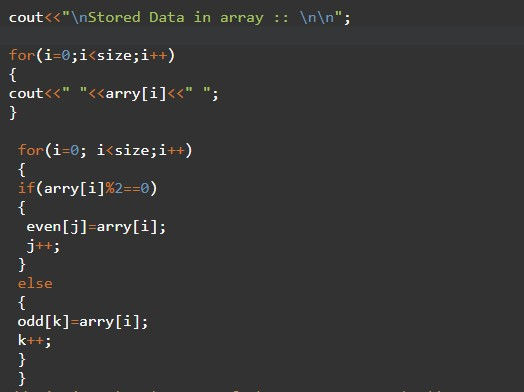Program Logic Code and print the even & odd numbers Separately.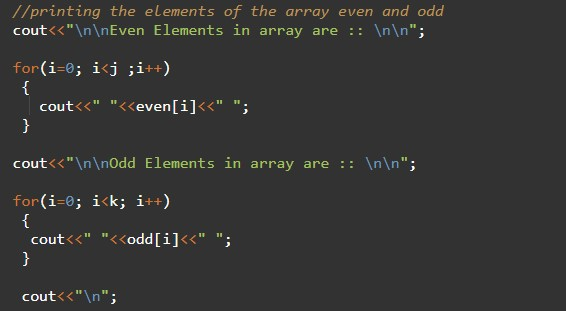In this tutorial we have learn about the C++ program to find even and odd elements in array and its application with practical example. I hope you will like this tutorial.